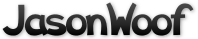Got questions, comments, patches, etc.? Contact Jason Woofenden
1 #include <math.h>
2 #include <stdlib.h>
3 #include <string.h>
4 #include "vorconfig.h"
5 #include "common.h"
6 #include "globals.h"
7 #include "sprite.h"
8 #include "rocks.h"
13 // 2 sets of sprites, sorted by position
14 static Sprite **sprites = { NULL, NULL };
16 // which set are we using?
17 static int set = 0;
19 // size of squares into which sprites are sorted.
20 static int grid_size = 0;
22 // screen size in grid squares.
23 static int gw = 0, gh = 0;
25 // lists of free sprites, by type.
26 Sprite *free_sprites[N_TYPES];
29 static void
30 get_shape(Sprite *s)
31 {
32         int x, y;
33         uint16_t *px, transp;
34         uint32_t bits = 0, bit, *p;
36         s->area = 0;
37         if(s->image->format->BytesPerPixel != 2) {
38                 fprintf(stderr, "get_shape(): not a 16-bit image!\n");
39                 exit(1);
40         }
42         s->w = s->image->w; s->h = s->image->h;
43         grid_size = max(grid_size, max(s->w, s->h));
47                 fprintf(stderr, "get_shape(): can't allocate bitmask.\n");
48                 exit(1);
49         }
51         if(SDL_MUSTLOCK(s->image)) { SDL_LockSurface(s->image); }
52         px = s->image->pixels;
53         transp = s->image->format->colorkey;
55         for(y=0; y<s->image->h; y++) {
56                 bit = 0;
57                 for(x=0; x<s->image->w; x++) {
58                         if(!bit) { bits = 0; bit = 0x80000000; }
59                         if(*px++ != transp) { bits |= bit; s->area++; }
60                         bit >>= 1;
61                         if(!bit || x == s->image->w - 1) { *(p++) = bits; }
62                 }
63                 px = (uint16_t *) ((uint8_t *) px + s->image->pitch - 2*s->image->w);
64         }
65         if(SDL_MUSTLOCK(s->image)) { SDL_UnlockSurface(s->image); }
66 }
69 void
71 {
73         if(s->image) get_shape(s);
74 }
77 static void
79 {
82 }
85 void
86 init_sprites(void)
87 {
90         grid_size = grid_size * 3 / 2;
91         gw = (XSIZE + 2*grid_size) / grid_size; // -grid-size to XSIZE inclusive (so sprites can be just off either edge)
92         gh = (YSIZE + 2*grid_size) / grid_size;
94         sprites = malloc(2 * gw * gh * sizeof(Sprite *));
95         sprites = (void *)sprites + gw * gh * sizeof(Sprite *);
96         if(!sprites) {
97                 fprintf(stderr, "init_sprites(): can't allocate grid squares.\n");
98                 exit(1);
99         }
100         memset(sprites, 0, 2 * gw * gh * sizeof(Sprite *));
101         set = 0;
102 }
104 static inline Sprite **
105 square(int x, int y, int set)
106 {
107         int b = (x+grid_size)/grid_size + gw*((y+grid_size)/grid_size);
108         if(b >= gw*gh || b < 0) {
109                 fprintf(stderr, "square(%i, %i, %i) = %i\n", x, y, set, b);
110                 ((int*)0) = 0;
111         }
112         return &sprites[set][b];
113 }
115 void
117 {
118         insert_sprite(square(s->x, s->y, set), s);
119 }
121 void
122 reset_sprites(void)
123 {
124         int i;
126         for(i=0; i<gw*gh; i++)
127                 while(sprites[set][i]) {
128                         Sprite *s = remove_sprite(&sprites[set][i]);
129                         insert_sprite(&free_sprites[s->type], s);
130                         s->flags = 0;
131                 }
132 }
134 void
135 move_sprite(Sprite *s)
136 {
137         if(s->flags & MOVE) {
138                 s->x += (s->dx - screendx)*t_frame;
139                 s->y += (s->dy - screendy)*t_frame;
140         }
141 }
143 void
144 sort_sprite(Sprite *s)
145 {
146         // clip it, or sort it into the other set of sprites.
147         if(s->x + s->w < 0 || s->x >= XSIZE
148            || s->y + s->h < 0 || s->y >= YSIZE) {
149                 insert_sprite(&free_sprites[s->type], s);
150                 s->flags = 0;
151         } else insert_sprite(square(s->x, s->y, 1-set), s);
152 }
154 void
155 move_sprites(void)
156 {
157         int sq;
160         // Move all the sprites
161         for(sq=0; sq<gw*gh; sq++) {
165                         move_sprite(s); sort_sprite(s);
166                 }
167         }
168         set = 1-set;  // switch to other set of sprites.
169 }
172 // xov: number of bits of overlap
173 // bit: number of bits in from the left edge of amask where bmask is
174 static int
176 {
177         int i, words = (xov-1) >> 5;
178         uint32_t abits;
180         for(i=0; i<words; i++) {
181                 abits = *amask++ << bit;
182                 abits |= *amask >> (32-bit);
183                 if(abits & *bmask++) return true;
184         }
185         abits = *amask << bit;
186         if(abits & *bmask) return true;
188         return false;
189 }
191 // xov: number of bits/pixels of horizontal overlap
192 // yov: number of bits/pixels of vertical overlap
193 static int
194 mask_collide(int xov, int yov, Sprite *a, Sprite *b)
195 {
196         int y;
197         int xoffset = a->w - xov;
198         int word = xoffset >> 5, bit = xoffset & 31;
201         if(yov > 0) {
204         } else {
205                 yov = -yov;
208         }
210         for(y=0; y<yov; y++) {
213         }
215         return 0;
216 }
218 int
219 collide(Sprite *a, Sprite *b)
220 {
221         int dx, dy, xov, yov;
223         if(!COLLIDES(a) || !COLLIDES(b)) return false;
225         if(b->x < a->x) { Sprite *tmp = a; a = b; b = tmp; }
227         dx = b->x - a->x;
228         dy = b->y - a->y;
230         xov = max(min(a->w - dx, b->w), 0);
232         if(dy >= 0) yov = max(min(a->h - dy, b->h), 0);
233         else yov = -max(min(b->h - -dy, a->h), 0);
235         if(xov == 0 || yov == 0) return false;
236         else return mask_collide(xov, yov, a, b);
237 }
239 void
240 collide_with_list(Sprite *s, Sprite *list)
241 {
242         for(; list; list=list->next)
243                 if(collide(s, list)) do_collision(s, list);
244 }
246 void
247 collisions(void)
248 {
249         int i, end = gw*gh;
250         Sprite *s;
251         for(i=0; i<end; i++) {
252                 for(s=sprites[set][i]; s; s=s->next) {
253                         collide_with_list(s, s->next);
254                         if(i+1 < end) collide_with_list(s, sprites[set][i+1]);
255                         if(i+gw < end) collide_with_list(s, sprites[set][i+gw]);
256                         if(i+gw+1 < end) collide_with_list(s, sprites[set][i+gw+1]);
257                 }
258         }
259 }
261 int
262 pixel_collide(Sprite *s, int x, int y)
263 {
266         if(!COLLIDES(s)) return false;
268         if(x < s->x || y < s->y || x >= s->x + s->w || y >= s->y + s->h) return 0;
270         x -= s->x; y -= s->y;
271         pmask = 0x80000000 >> (x&0x1f);
273 }
275 Sprite *
276 pixel_hit_in_square(Sprite *r, float x, float y)
277 {
278         for(; r; r=r->next) {
279                 if(COLLIDES(r) && pixel_collide(r, x, y)) return r;
280         }
281         return 0;
282 }
284 Sprite *
285 pixel_collides(float x, float y)
286 {
287         int l, t;
288         Sprite **sq;
289         Sprite *ret;
291         l = (x + grid_size) / grid_size; t = (y + grid_size) / grid_size;
292         sq = &sprites[set][l + t*gw];
293         if((ret = pixel_hit_in_square(*sq, x, y))) return ret;
294         if(l > 0 && (ret = pixel_hit_in_square(*(sq-1), x, y))) return ret;
295         if(t > 0 && (ret = pixel_hit_in_square(*(sq-gw), x, y))) return ret;
296         if(l > 0 && t > 0 && (ret = pixel_hit_in_square(*(sq-1-gw), x, y))) return ret;
297         return 0;
298 }
301 float
302 sprite_mass(Sprite *s)
303 {
304         if(s->type == SHIP) return s->area;
305         else if(s->type == ROCK) return 3 * s->area;
306         else return 0;
307 }
309 /*
310  * BOUNCE THEORY
311  *
312  * ******************  In 1 Dimension  *****************
313  *
314  * For now we will imagine bouncing A and B off each other in 1 dimension (along
315  * a line). We can safely save the other dimension for later.
316  *
317  * A and B are the same weight, and are both traveling 1m/sec, to collide right
318  * at the origin. With perfect bounciness, their full momentum is reversed.
319  *
320  * If we cut the weight of A down by half, then the center of our colision will
321  * drift towards A (the speeds of A and B are not simply reversed as in our last
322  * example.) However, there is always a place between A and B on the line (I'll
323  * call it x) such that the speeds of A and B relative to x, are simply
324  * reversed. Thus we can find the new speed for A like so:
325  *
326  *     new A = x -(A - x)
327  *
328  *     new B = x -(B - x)
329  *
330  * or, simply:
331  *
332  *     new A = 2x - A
333  *
334  *     new B = 2x - B
335  *
336  *
337  * this point x is the sort of center of momentum. If, instead of bouncing, A
338  * and B just globbed together, x would be center of the new glob.
339  *
340  * x is the point where there's an equal amount of force coming in from both
341  * sides. ie the weighted average of the speeds of A and B.
342  *
343  * average force = (A force + B force) / total mass
344  *
345  * x.speed = (a.speed * a.mass + b.speed * b.mass) / (a.mass + b.mas)
346  *
347  * then we apply the formula above for calculating the new A and B.
348  *
349  *
350  *
351  *
352  * ******************  In 2 Dimensions  *****************
353  *
354  * OK, that's how we do it in 1D. Now we need to deal with 2D.
355  *
356  * Imagine (or draw) the two balls just as they are bouncing off each other.
357  * Imagine drawing a line through the centers of the balls. The balls are
358  * exerting force on each other only along this axis. So if we rotate
359  * everything, we can do our earlier 1D math along this line.
360  *
361  * It doesn't matter what direction the balls are going in, they only exert
362  * force on each other along this line. What we will do is to compute the part
363  * of the balls' momentum that is going along this line, and bounce it according
364  * to our math above. The other part is unaffected by the bounce, and we can
365  * just leave it alone.
366  *
367  * To get this component of the balls' momentum, we can use the dot product.
368  *
369  *     dot(U, V) = length(U) * length(V) * cos(angle between U and V)
370  *
371  * If U is a length 1 vector, then dot(U, V) is the length of the component of V
372  * in the direction of U.  So the components of V are:
373  *
374  *     U * dot(U, V)      parallel to U
375  *
376  *     V - U * dot(U, V)  perpendicular to U
377  *
378  * To do the actual bounce, we compute the unit vector between the center of the
379  * two balls, compute the components of the balls' speeds along this vector (A
380  * and B), and then bounce them according to the math above:
381  *
382  *     new A = 2x - A
383  *
384  *     new B = 2x - B
385  *
386  * But we rewrite it in relative terms:
387  *
388  *     new A = A + 2(x-A)
389  *
390  *     new B = B + 2(x-B)
391  */
393 void
394 bounce(Sprite *a, Sprite *b)
395 {
396         float x, y, n;  // (x, y) is unit vector from a to b.
397         float va, vb;   // va, vb are balls' speeds along (x, y)
398         float ma, mb;   // ma, mb are the balls' masses.
399         float vc;       // vc is the "center of momentum"
401         // (x, y) is unit vector pointing from A's center to B's center.
402         x = (b->x + b->w / 2) - (a->x + a->w / 2);
403         y = (b->y + b->h / 2) - (a->y + a->h / 2);
404         n = sqrt(x*x + y*y); x /= n; y /= n;
406         // velocities along (x, y)
407         va = x*a->dx + y*a->dy;
408         vb = x*b->dx + y*b->dy;
409         if(vb-va > 0) return;  // don't bounce if we're already moving away.
411         // get masses and compute "center" speed
412         ma = sprite_mass(a); mb = sprite_mass(b);
413         vc = (va*ma + vb*mb) / (ma+mb);
415         // bounce off the center speed.
416         a->dx += 2*x*(vc-va); a->dy += 2*y*(vc-va);
417         b->dx += 2*x*(vc-vb); b->dy += 2*y*(vc-vb);
418 }